Study Materials: ncert solutions

Our ncert solutions for Exercise 7.1 Class 9 maths 7. Triangles - ncert solutions - Toppers Study is the best material for English Medium students cbse board and other state boards students.

Solutions ⇒ Class 9th ⇒ Mathematics ⇒ 7. Triangles

# Exercise 7.1 Class 9 maths 7. Triangles - ncert solutions - Toppers Study

Topper Study classes prepares ncert solutions on practical base problems and comes out with the best result that helps the students and teachers as well as tutors and so many ecademic coaching classes that they need in practical life. Our ncert solutions for Exercise 7.1 Class 9 maths 7. Triangles - ncert solutions - Toppers Study is the best material for English Medium students cbse board and other state boards students.

## Exercise 7.1 Class 9 maths 7. Triangles - ncert solutions - Toppers Study

CBSE board students who preparing for class 9 ncert solutions maths and Mathematics solved exercise chapter 7. Triangles available and this helps in upcoming exams 2023-2024.

### You can Find Mathematics solution Class 9 Chapter 7. Triangles

• All Chapter review quick revision notes for chapter 7. Triangles Class 9
• NCERT Solutions And Textual questions Answers Class 9 Mathematics
• Extra NCERT Book questions Answers Class 9 Mathematics
• Importatnt key points with additional Assignment and questions bank solved.

NCERT Solutions do not only help you to cover your syllabus but also will give to textual support in exams 2023-2024 to complete exercise 7.1 maths class 9 chapter 7 in english medium. So revise and practice these all cbse study materials like class 9 maths chapter 7.1 in english ncert book. Also ensure to repractice all syllabus within time or before board exams for ncert class 9 maths ex 7.1 in english.

See all solutions for class 9 maths chapter 7 exercise 7 in english medium solved questions with answers.

### Exercise 7.1 class 9 Mathematics Chapter 7. Triangles

• Exercise 7.1 Class 9 Maths 7. Triangles - Ncert Solutions - Toppers Study
• Class 9 Ncert Solutions
• Solution Chapter 7. Triangles Class 9
• Solutions Class 9
• Chapter 7. Triangles Exercise 7.1 Class 9

## Exercise 7.1 Class 9 maths 7. Triangles - ncert solutions - Toppers Study

Chapter 7. Triangles

Exercise 7.1

Q1. In quadrilateral ACBD, AC = AD and AB bisects ∠A (see Fig. 7.16). Show that Δ ABC ≅ Δ ABD.Solution:

Given: AC = AD and AB bisects ∠A

To prove:  Δ ABC ≅ Δ ABD.

Proof: In Δ ABC and Δ ABD.

∠CAB = ∠BAD   [AB bisect ∠A]

AB = AB         [Common]

By SAS Congruence Criterion Rule

Δ ABC ≅ Δ ABD

BC = BD [By CPCT]   Proved

Q2. ABCD is a quadrilateral in which AD = BC and ∠ DAB = ∠ CBA (see Fig.7.17). Prove that

(i) Δ ABD ≅ Δ BAC

(ii)   BD = AC

(iii)  ∠ ABD = ∠ BAC

Solution:

Given: ABCD is a quadrilateral in which AD = BC and ∠ DAB = ∠ CBA

To prove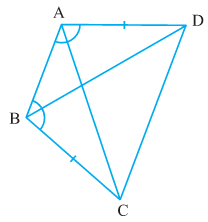(i) Δ ABD ≅ Δ BAC

(ii)   BD = AC

(iii)  ∠ ABD = ∠ BAC

Proof:  (i) In Δ ABD and Δ BAC

∠ DAB = ∠ CBA   [given]

AB = AB         [Common]

By SAS Congruency Criterion Rule

Δ ABD ≅ Δ BAC

(ii)                    BD = AC [CPCT]

(iii)            ∠ ABD = ∠ BAC    [CPCT]

Q3.  AD and BC are equal perpendiculars to a line segment AB (see the given figure). Show that CD bisects AB.

Solution: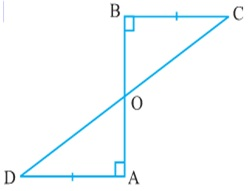Given: AD and BC are equal perpendiculars to a line segment AB.

To prove: CD bisects AB.

Proof:

In ∆BOC and ∆AOD

∠ BOC = ∠AOD (Vertically opposite angles)

∠CBO = ∠DAO (Each 90º)

By AAS Congruence Criterion Rule

∆BOC ≅ ∆AOD

BO = AO (By CPCT)

Hence, CD bisects AB.

Q4. l and m are two parallel lines intersected by another pair of parallel lines p and q (See the given figure). Show that ∆ABC ≅ ∆CDA

Solution: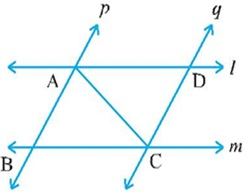Given: l and m are two parallel lines intersected by another pair of   parallel lines p and q.

To prove: ∆ABC ≅ ∆CDA

Proof:

In ∆ABC and ∆CDA,

∠ BAC = ∠DCA (Alternate interior angles, as p || q)

AC = CA (Common)

∠ BCA = ∠DAC (Alternate interior angles, as l || m)

By AAS Congruence Criterion Rule

∆ABC ≅ ∆CDA

Q5. Line l is the bisector of an angle ∠A and B is any point on l. BP and BQ are perpendiculars from B to the arms of a (see the given figure). Show that: (i) ∆APB ≅ ∆AQB (ii) BP = BQ or B is equidistant from the arms of ∠A.

Solution:

Given: Line l is the bisector of an angle ∠A and B is any point on l. BP and BQ are perpendiculars from B to the arms of a.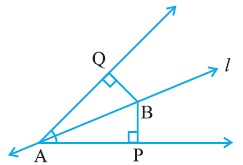To prove:

(i) ∆APB ≅ ∆AQB

(ii) BP = BQ or B is equidistant from the arms of ∠A.

Proof:

In ∆APB and ∆AQB,

∠ APB = ∠AQB (Each 90º)

∠ PAB = ∠QAB (l is the angle bisector of A)

AB = AB (Common)

By AAS Congruence Criterion Rule

∆APB ≅ ∆AQB

BP = BQ    [CPCT]

it can be said that B is equidistant from the A.

Q6. In the given figure, AC = AE, AB = AD and ∠BAD = ∠EAC. Show that BC = DE.

Solution: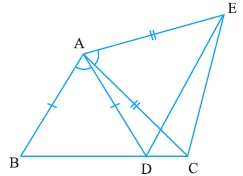To prove: BC = DE.

BAD + DAC = EAC + DAC

BAC = DAE

In ∆BAC and ∆DAE

AC = AE (Given)

∠BAC = ∠DAE (proved above)

By SAS Congruence Criterion Rule

∆BAC ≅ ∆DAE

BC = DE (CPCT)

Q7.  AB is a line segment and P is its mid-point. D and E are points on the same side of AB such that ∠BAD =∠ ABE and ∠EPA = ∠DPB (See the given figure).

Show that:(i) ∆DAP ≅ ∆EBP

Solution:

Given: AB is a line segment and P is its mid-point. D and E are points on the same side Of AB such that ∠BAD =∠ ABE and ∠EPA = ∠DPB.

To prove:

(i) ∆DAP ≅ ∆EBP

Proof:  In ∆ DPA and ∆ EPB

∠EPA = ∠DPB

EPA + DPE = DPB + DPE

∠ DPA = ∠EPB

∠EPA = ∠DPB (Given)

AP =BP (P is the midpoint of AB)

By AAS Congruence Criterion Rule

∆DAP ≅ ∆EBP

##### Other Pages of this Chapter: 7. Triangles

Important Study materials for classes 06, 07, 08,09,10, 11 and 12. Like CBSE Notes, Notes for Science, Notes for maths, Notes for Social Science, Notes for Accountancy, Notes for Economics, Notes for political Science, Noes for History, Notes For Bussiness Study, Physical Educations, Sample Papers, Test Papers, Mock Test Papers, Support Materials and Books.Mathematics Class - 11th

NCERT Maths book for CBSE Students.

books

## Study Materials List:

##### Solutions ⇒ Class 9th ⇒ Mathematics
1. Number Systems
2. Polynomials
3. Coordinate Geometry
4. Linear Equation In Two Variables
5. Introduction To Euclid’s Geometry
6. Lines and Angles
7. Triangles